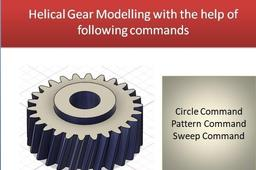# Helical gear in fusion 360In this Tutorial we create a helical gear with the help of following command:
Circle command
Line Command
Tangent constraint
Pattern command
Sweep command
also discussed about terminology used in helical gear.
for terminology of gear you can click on below link of spur gear.
Terminology used for gear are as follows
Module(m) =PCD/No. of teeth=10
No of teeth(N) =26
PCD = m X no. of teeth = 10X26=260
Outside dia= PCD+2Xadd = 260+20 =280mm
Inside dia = PCD -2 X Ded = 260 -2 X11.25= 237.5 mm
Circular pitch = π X PCD /N = π X 260/26=31.4mm
Tooth thickness = CP/2= 31.4/2 = 15.7 mm
Pressure Angle = 20 degree
Helix angle = 10 degree

1. ### Step 1:

Dear viewers,

follow the process to create helical gear as discussed in video. you are able to create any type of gear after watching the video.